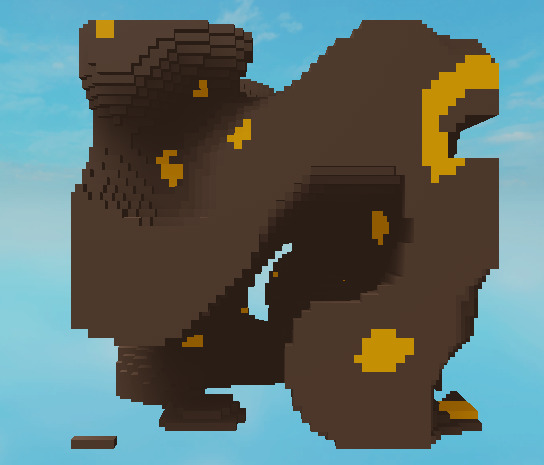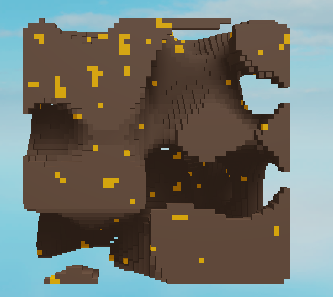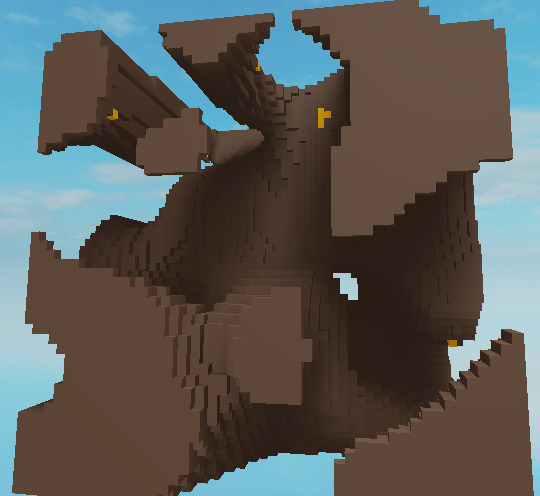# How could I creat a random generated cave system? (NOT INF)

I would like to create a large chunk of blank stone, with a cave system inside of it. I’ve heard about using Perlin noise, but I have no idea where to start, and how to break that down into code. Its gonna be a system like minecraft, but only underground without going above ground. I only need it around a 500,200,500 stud scale. Thank you for any responses or feedback! Also please demonstrate like im 5 because im very new to this and I don’t understand italso heres a script generation that i borrowed from Realistic Cave Generation. it generates terrain on the ground, but not underground. also put this in a “script” in server script service and add a part of any scale to workspace.

``````p.Anchored = true;

local folder = Instance.new'Folder';
folder.Parent = workspace;
folder.Name = 'Parts';

local f = 67
local coeff = 450;

function calc(x,z,o)
local height = 0;
local r = math.random(8,8*2);
for i = 1, r do
height = height + math.noise(x/(coeff/i)+o,z/(coeff/i)+o);
end
height = height/r;
return height;
end

for i = 1, 450 do
for j = 1, 450 do
local new = p:Clone();
new.Parent = folder;
new.Position = Vector3.new(i*new.Size.x, 0+coeff*calc(i,j,f), j*new.Size.z);
end
end

2 Likes

Perlin worms can really complicate a chunk-based infinite terrain generator, but since you don’t care about infinite terrain then they’re an okay solution. One alternative is certain noise functions that can be tuned to spit out similar caves. For starters though, just get down the basics of generating 3D terrain.

This video is pretty great: Minecraft terrain generation in a nutshell - YouTube

Here’s a minimal starter example:

``````local size = Vector3.new(100, 100, 100)
local scale = script.Block.Size.X

local threshold = 0
local seed = Random.new():NextInteger(0, 10e6)
local frequency = 1/16

function density(p: Vector3): number
return math.noise(
p.X * frequency,
p.Y * frequency,
p.Z * frequency
)
end

for x = 1, size.X do
for y = 1, size.Y do
if y%10 == 0 then task.wait() end
for z = 1, size.Z do
local d = density(Vector3.new(x + seed, y, z))
if d < threshold then continue end

local b = script.Block:Clone()
b.CFrame = CFrame.new(x*scale, y*scale, z*scale)
b.Parent = game.Workspace.Blocks
end
end
end
``````

1 Like

Create loads of different cave parts and turn them into models. Then put them in 1 folder.

Here is a code snippet to make the game choose one of these models:

``````local folder = --folder location
local chosenModel
for _,v in pairs(folder:GetChildren()) do
if math.random(1,10) == 5 then
chosenModel = v
end
end
``````

Then you would write some code to place the model, put all the picking and placing code in a loop, and there ya go!

Thank you! This works pretty good, but could you explain some of the code so that I can change it later on? (im super new)

1 Like

Sure! It loops over every voxel aka block position and decides if it should place a block there or not (e.g. “is it stone or air”). This is decided by evaluating a coherent noise function at each position, which is a function that varies smoothly for inputs that are close together but looks completely random for inputs that are far together. This gives the smooth look to the caves at a small scale, but random variation at a larger scale. Blocks are placed at a position if the function is above some threshold when evaluated at that position.

The specific coherent noise function is called Perlin noise and is built into Roblox as the `math.noise` function. This specific implementation takes up to 3 numbers and outputs 1 number. The input difference-scale at which output appears random for this function is 1, so we only get smooth outputs if we evaluate points that are less than 1 from each other. At each iteration of the loop, the current block position is described by `x`, `y` and `z`, which are passed to the function. The output is compared to the threshold to see if a block should be placed.

The actual function that’s evaluated isn’t just math.noise, but one where the domain is scaled (multiplied) by a constant in each dimension. Since it’s scaled down, we end up evaluation points that are closer together and we thus get smooth results.

A random “seed” is added to the input X coordinate so we get different results each time, but note that this isn’t a great way since it really just shifts the terrain around.

`if y%10 == 0 then task.wait() end`

This just makes the script yield once every 10 Y layers so it doesn’t crash or w/e.

1 Like

Oh, and check out this glossary for an overview of common terms used to talk about Perlin and other noise functions: libnoise: Glossary

1 Like

I know I’m asking a lot of questions, but how could I add multiple different ores that spawn, for instance every 100 blocks is a vein of 4 different ores. I don’t know if this would even be possible though. Thank you for everything, your a life saver!

1 Like

You could spread blobs of ore around using e.g. Poisson disc (sphere) sampling: Poisson-Disc Sampling

It has the same problem as Perlin worms, that to generate any given block you need to know what worms or ore blobs might intersect that block. In practice that means you have to generate worms/blobs further out than the frontier of actually-generated blocks, so you now have multiple phases of generating and that’s just more complexity.

A more common approach is to use a different noise function or even lots of noise functions to determine what each block type should be.

Here are some example results you can get by tuning the frequency and threshold of a “metalicity” noise function:

freq 1/8, thresh 0.5:freq 1/3, thresh 0.5freq 1/3, thresh 0.7Here's the code for that
``````local size = Vector3.new(50, 50, 50)
local scale = script.Dirt.Size.X

local rng = Random.new()
local seed = rng:NextInteger(-103, 10e3)

--Returns a noise function with a given seed and frequency
function newNoise(seed: number, frequency: number)
local noise = math.noise
return function(p: Vector3): number
return noise(
seed + p.X * frequency,
seed + p.Y * frequency,
seed + p.Z * frequency
)
end
end

--Takes a function f and returns a function f' that returns whether f returns a number >= threshold for any given input
function thresholded(f, threshold)
return function(...): boolean
return f(...) >= threshold
end
end

local density = newNoise(seed, 1/16)
local isSolid = thresholded(density, 0)

local metalicity = newNoise(-seed, 1/3)

function blockType(p: Vector3): Model
return metalicity(p) > 0.7 and script.Gold or script.Dirt
end

for x = 1, size.X do
for y = 1, size.Y do
if y%10 == 0 then task.wait() end
for z = 1, size.Z do
local p = Vector3.new(x, y, z)
if not isSolid(p) then continue end

local b = blockType(p):Clone()
b:PivotTo(CFrame.new(x*scale, y*scale, z*scale))
b.Parent = game.Workspace.Blocks
end
end
end
``````

There is soooo much you can play with with this approach. For example you could vary the metalicity threshold as the Y coordinate/depth varies, or even use yet another noise function to control the threshold.

1 Like

Yes! You’re very smart. I only have 1 more question, I swear. How could I make the terrain generation larger without it being soo laggy. I’m trying to make a 200 x 200 x 200 chunk, but it freezes studio, and I have a very powerful computer too. Is there a way to load this in multiple chunks like minecraft?

1 Like

Chunk loading is one possible implementation, but there are others. An important one is to distinguish between outside an inside the terrain, if your game design supports it. That way, you can get away with only actually creating blocks on the very boundary of the terrain, potentially saving 100s, 1000s or even 10000s of blocks being created. Of course this means additional blocks have to be created/destroyed if adjecent blocks are mined/built.

EDIT: As for how to implement chunk loading, the basic idea is to round/snap each player’s position to the nearest `chunk_size`, e.g. 2*16 for blocks of size 2 and chunks of size 16, and then looping over every chunk position in range to determine which chunks to generate.

The reason im tryng to do chunks, is to improve the generation speed and the amount of lag. Because when I generate a 50,50,50 chunk, it generates very fast, but when I generate a 100,100,100 chunk, it generates a lot slower. I don’t really need a loading method though, because the map will only be around 500x500x500 studs wide. But just for less laggy and faster generation. Is there a way I could do this?

Generating in chunks wouldn’t make a difference for that, since you still have to make the same number of blocks. Try making the loop wait like this instead:

``````local speed = 100
local count = 0
for x = 1, size.X do
for y = 1, size.Y do
for z = 1, size.Z do
...
if count % speed == 0 then task.wait() end
count += 1
end
end
end
``````

Lower the speed if you get crashes

This topic was automatically closed 14 days after the last reply. New replies are no longer allowed.